# CLASS 8 MATHS CHAPTER -10 VISUALISING SOLID SHAPES

### Exercise 10.1

Question 1.

For each of the given solid, the two views are given. Match for each solid the corresponding top and front views. The first one is done for you.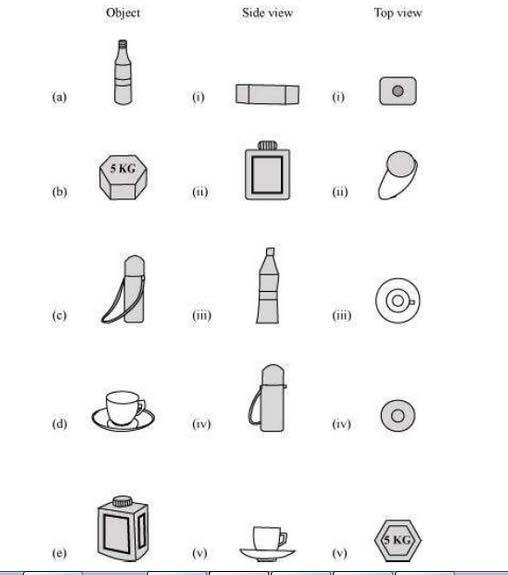Solution :

(a)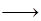(iii);(iv)
(b);(i);; (v)
(c);(iv)(ii)
(d)(v);(iii)
(e)(ii);(i)
;

Question 2.

For each of the given solid, the three views are given. Identify for each solid the corresponding top, front and side views.
;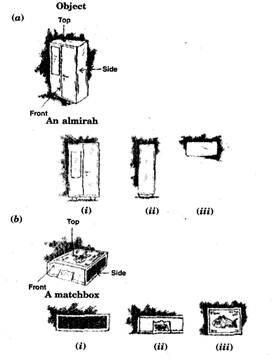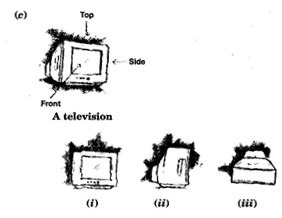Solution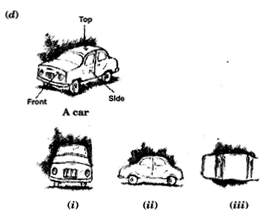:

(a);(i); Front
(ii)Side
(iii)Top view
(b)(i); Side
(ii)Front
(iii); Top view
(c)(i); Front
(ii);Side
(iii)Top view
(d)(i); Front
(ii);Side
(iii);Top view

Question 3.

For each given solid, identify the top view, front view and side view.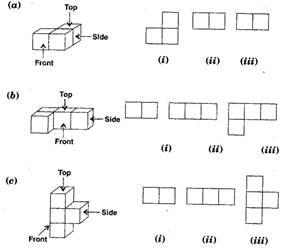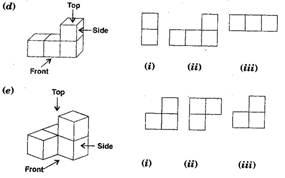Solution :

(a);(i)Top view(ii);Front view(iii);Side view
(b)(i)Side view(ii)Front view(iii);Top view
(c);(i);Top view(ii); Side view(iii)Front view
(d);(i)Side view(ii)Front view(iii)Top view
(e)(i);Front view(ii); Top view(iii)Side view

Question 4.

Draw the front view, side view and top view of the given objects: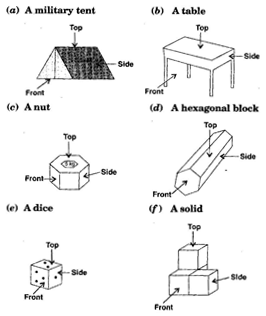Solution :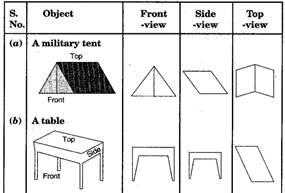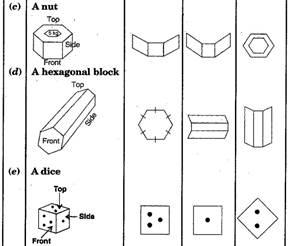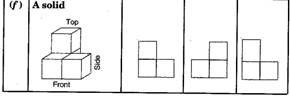### NCERT Solutions for Class 8 Maths Exercise 10.2

Question 1.

Can a polygon have for its faces:
(i) 3 triangles ;
(ii) 4 triangles
(iii) a square and four triangles

Solution :

(i) No, a polyhedron cannot have 3 triangles for its faces.
(ii) Yes, a polyhedron can have four triangles which is known as pyramid on triangular base.
(iii) Yes, a polyhedron has its faces a square and four triangles which makes a pyramid on square base.

Question 2.

Is it possible to have a polyhedron with any given number of faces? (Hint: Think of a pyramid)

Ans.

It is possible, only if the number of faces are greater than or equal to 4.

Question 3.

Which are prisms among the following: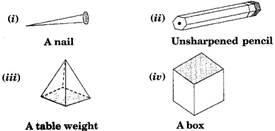Solution :

Figure (ii) unsharpened pencil and figure (iv) a box are prisms.

Question 4.

(i) How are prisms and cylinders alike?
(ii) How are pyramids and cones alike?

Solution :

(i) A prism becomes a cylinder as the number of sides of its base becomes larger and larger.
(ii) A pyramid becomes a cone as the number of sides of its base becomes larger and larger.

Question 5.

Is a square prism same as a cube? Explain.

Solution :

No, it can be a cuboid also.

Question 6.

Verify Euler’s formula for these solids.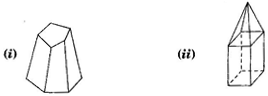Solution :

(i) Here, figure (i) contains 7 faces, 10 vertices and 15 edges.
Using Eucler’s formula, we see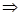F + V – E = 2
Putting F = 7, V = 10 and E = 15,F + V – E = 27 + 10 – 5 = 217 – 15 = 22 = 2L.H.S. = R.H.S.
(ii); Here, figure (ii) contains 9 faces, 9 vertices and 16 edges.
Using Eucler’s formula, we see
F + V – E = 2
F + V – E = 29 + 9 – 16 = 218 – 16 = 22 = 2L.H.S. = R.H.S.
Hence verified Eucler’s formula.

Question 7.

Using Euler’s formula, find the unknown:

Solution :

In first column, F = ?, V = 6 and
E = 12
Using Eucler’s formula, we see
F + V – E = 2
F + V – E = 2
;F + 6 – 12 = 2
;F – 6 = 2
;F = 2 + 6 = 8
Hence there are 8 faces.
In second column, F = 5, V = ? and E = 9
Using Eucler’s formula, we see
F + V – E = 2
F + V – E = 2
;5 + V – 9 = 2
;V – 4 = 2
;V = 2 + 4 = 6
Hence there are 6 vertices.
In third column, F = 20, V = 12 and E = ?
Using Eucler’s formula, we see
F + V – E = 2
F + V – E = 2
;20 + 12 – E = 2
;32 – E = 2
;E = 32 – 2 = 30
Hence there are 30 edges.

Question 8.

Can a polyhedron have 10 faces, 20 edges and 15 vertices?

Solution :

If F = 10, V = 15 and E = 20.
Then, we know Using Eucler’s formula,
F + V – E = 2
L.H.S. = F + V – E
= 10 + 15 – 20
= 25 – 20
= 5
R.H.S.; = 2
Hence  L.H.S. R.H.S.
Therefore, it does not follow Eucler’s formula.
So polyhedron cannot have 10 faces, 20 edges and 15 vertices.

### NCERT Solutions for Class 8 Maths Exercise 10.3

Get 30% off your first purchase!

X
error: Content is protected !!
Scroll to Top